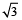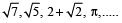# SCC Education

## Types of Numbers( Real numbers,Natural numbers,Whole numbers etc)

### Types of Numbers

Real numbers: Real numbers comprise the full spectrum of numbers.
They can take on any form – fractions or whole numbers, decimal points
or no decimal points. The full range of real numbers includes decimals
that can go on forever and ever without end.
For Example: 8, 6, 2 +, 3/5 etc.
Natural numbers: A natural number is a number that comes naturally.
Natural Numbers are counting numbers from 1, 2, 3, 4, 5, ……..

Whole numbers: whole numbers are just all the natural numbers plus zero.
For Example: 0, 1, 2, 3, 4, 5, and so on up to infinity.
Integers: Integers incorporate all the qualities of whole numbers and their opposites
(or additive inverses of the whole numbers) .
Integers can be described as being positive and negative whole numbers.
For Example: … –3, –2, –1, 0, 1, 2, 3, . . .
Rational Numbers: All numbers of the form p/q where p and q are integers
(q ≠ 0) called Rational numbers.
For Example: 4, 3/4, 0, ….
Irrational Numbers: Irrational numbers are the opposite of rational numbers.
An irrational number cannot be written as a fraction,
and decimal values for irrational numbers never end and do not have a repeating
pattern in them. ‘pi’ with its never-ending decimal places, is irrational.
For Example:Even Numbers: An even number is
one that can be divided evenly by two leaving no remainder, such as 2, 4, 6, and 8.
Odd Numbers: An odd number is one that does not divide evenly by two,
such as 1, 3, 5, and 7.
Prime Number: A prime number is a number which can be divided only by 1 and itself.
The prime number has only two factors, 1 and itself.
For example: 2, 3, 7, 11, 13, 17, …. are prime numbers.
Composite Number: A Composite Number is a number which can be divided evenly.
Any composite number has additional factors than 1 and itself.
For example: 4, 6, 8, 9, 10 …..
Co-primes or Relatively prime numbers: A pair of numbers not having any common
factors other than 1 or –1. (Or alternatively their greatest common factor is 1 or –1)
For Example: 15 and 28 are co-prime, because the factors of 15 (1,3,5,15) , and the factors
of 28 (1,2,4,7,14,28) are not in common (except for 1) .
Twin Primes: A pair of prime numbers that differ by 2 (successive odd numbers that are
both Prime numbers) .
For Example: (3,5) , (5,7) , (11,13) , …

competitive exam

ssceducation,scc,education,free notes ,hindi medium notes sharma sir ,9718041826,eco,kuch bhi,ctet,sigma,flower ,on a ,science in hindi ,formula sheet ,notes in pdf ,fee education,maths, science3 ,physics in hindi, general science in hindi,best tutor, 5 star rating center ,grade a ,cbse notes ,